WEBINAR
Numerical Analysis of Magnetic Pulse Welding Process
Thursday, October 5, 2023
Time
SESSION 1
SESSION 2
CEST (GMT +2)
03:00 PM
08:00 PM
EDT (GMT -4)
09:00 AM
02:00 PM
HOME / Applications / Electro-Dynamic Analysis of a Voice Coil Actuator using EMS and SOLIDWORKS Motion

# Electro-Dynamic Analysis of a Voice Coil Actuator using EMS and SOLIDWORKS Motion

Used Tools:## Problem description

In this article, a voice coil actuator will be studied . A series of simulations will be performed using the optimal actuator parameters determined in .  In the first section, static analysis will be used to compute the Lorentz force of the coil versus both current and actuator positions. Simulation results will be compared to experimental data published in . Electrodynamic simulation will be used at the end to compute mechanical output data such linear displacement, speed and acceleration of the actuator.

EMS, SOLIDWORKS and SOLIDWORKS Motion will be used to carry out the different simulations of the studied voice coil actuator. Figures 1a), 1b) and 1c) show full and cross section views of the studied voice coil device. A stranded coil, made of copper with 760 turns, is free to move axially along the airgap zone created between the ferromagnetic shell and the permanent magnet. The neodymium permanent magnet N42, which is stationary and axially magnetized, is a second source of energy. both the orientor and shell are made of soft iron characterized by its high permeability. The flux orientor is used to guide the field and improve the magnetic circuit path. A bobbin is inserted to support of the coil.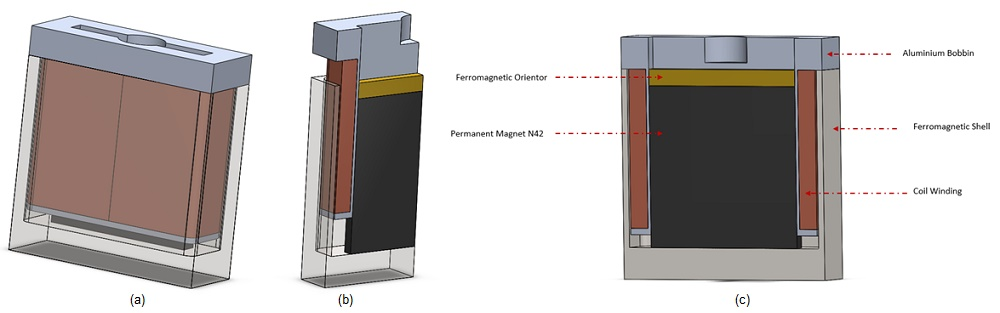Figure 1 - The studied voice coil actuator, a) full model, b) cross section view, c) agenda

## Simulation and Results

### Static Simulation -Geometrical Parametric Sweep

First simulations are performed at different DC current rates while changing the position of the moving parts (coil and bobbin). A parametric sweep study is defined to compute the magnetic flux, Lorentz force, the coil parameters, etc. Figures 2a) and 2b) display respectively full and cross section views of the magnetic flux density plots when the applied current is + 10A and the position of the assembly coil and bobbin is 10mm. The average of the magnetic flux is about [1.3T,1.74T]. Front and side cross section views of the magnetic field vector plot are shown in Figure 3a) and 3b). the current density of the coil is 1e8A/m^2.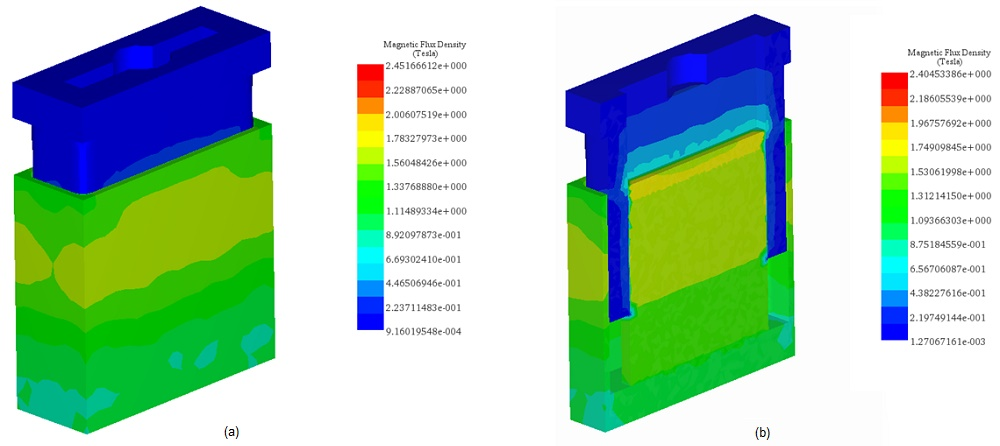Figure 2 - Fringe plot of the magnetic flux density, a) full model, b) cross vection view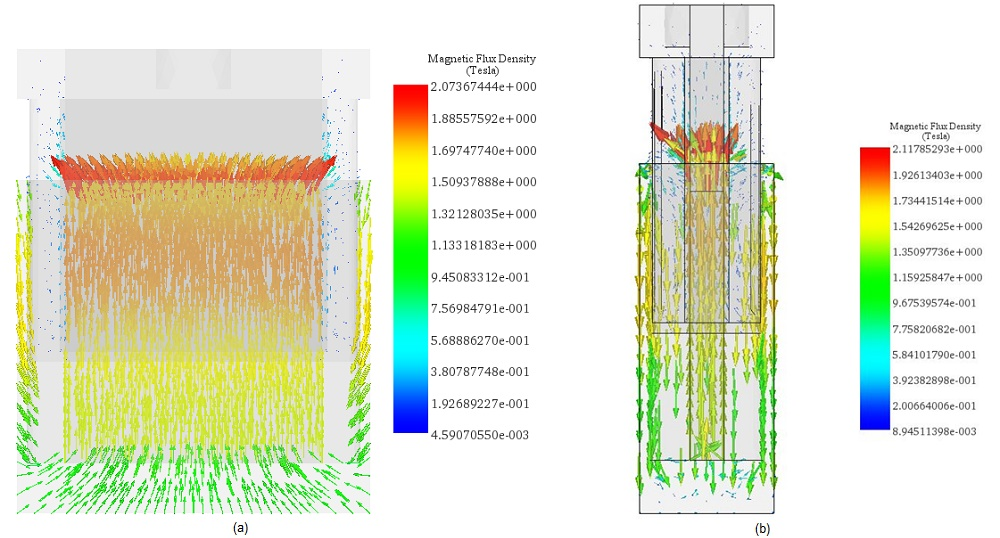Figure 3 - Vector plots of the magnetic flux density, a) front cross section view (+10A), b) side cross section view (-10A)

Figure 4 shows exprimental  and simulation results of the Lorentz force computed at both different DC currents and stroke values. The maximum force ( around 33N) is generated with a current of -10A at 10mm positon. The measured force values from 4 to 16 mm stroke can be considered as constant for the different applied current rates. Constant force rates with larger stroke can help to obtain a higher acceleration. Figure 5 contains a plot of the force peak values for different currents. It demonstrates that the force has a proportional linear relation with the current. This can illustrate the simple and flexible control of the actuator force density.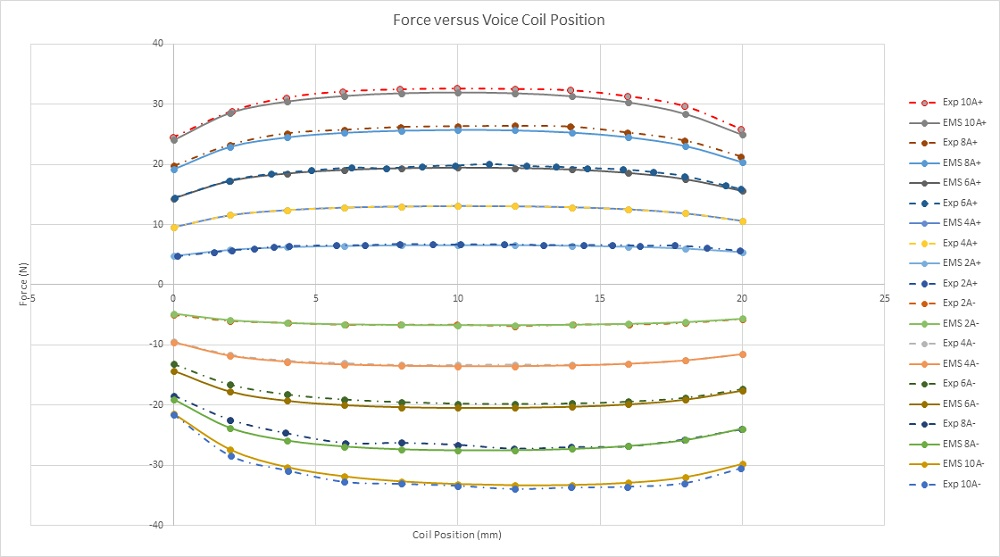Figure 4 - Force results versus coil position and applied current rates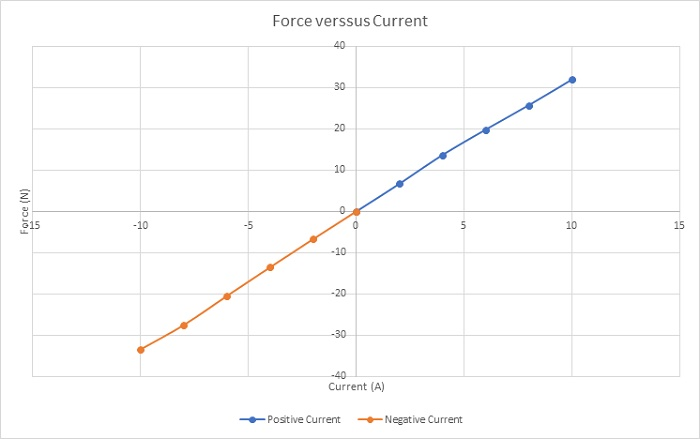Figure 5 - Peak values of the force versus applied current rates

Figure 6 gives the force sensitivity parameter for different input currents along the stroke. This parameter is constant regarding the current and depends only on the coil position. The simulated average value of the force sensitivity parameter (computed by EMS) is about 3.2N/amp which is close the experimental measured value (Exp 3.1N/amp).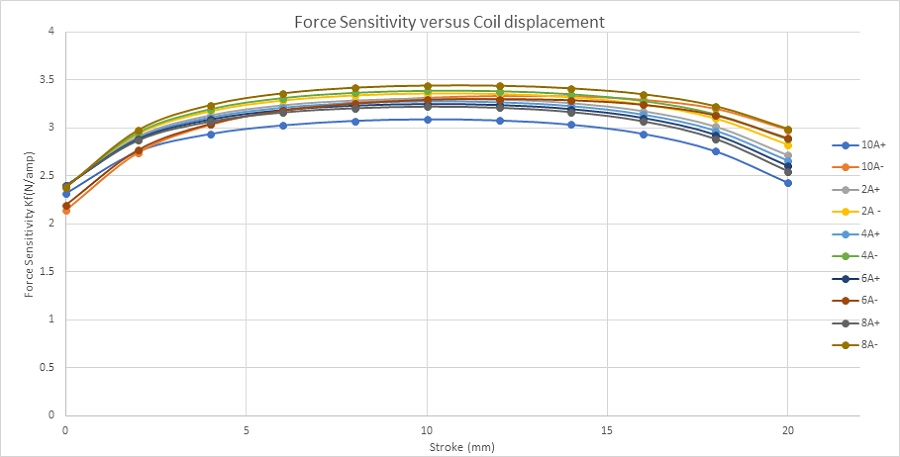Figure 6 - Force sensitivity parameter

Figure 7 shows the inductance curve versus coil displacement. The inductance decreases from 5mH at 0mm to 4.48mH at 20mm.The variation can be neglected, and the coil inductance can be considered as constant along the stroke.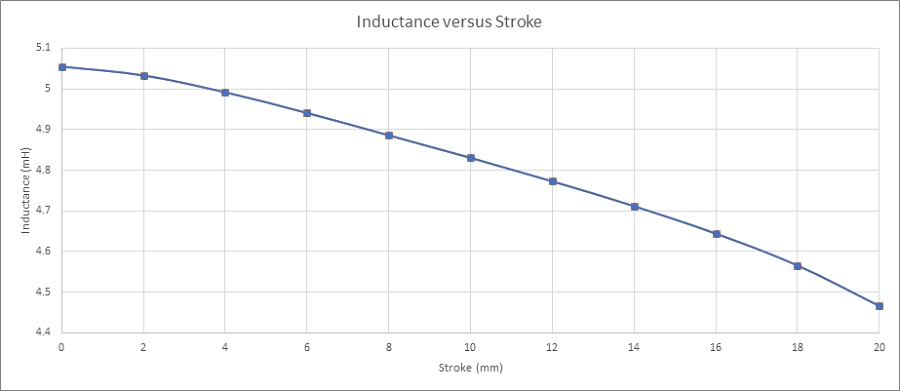Figure 7 - Coil inductance versus stroke

Figure 8 shows the Back emf results of the coil for different velocities and versus the center of mass of the moving components. It demonstrates that the Back emf increases with the speed. With a speed of 2m/s, it reaches a peak of 6.25V. Figure 9 contains the computed Back emf sensitivity parameter (V/speed) versus the coil position. It is a speed-constant parameter, i.e. it doesn’t change with the speed of the coil. It can be averaged to 3.1 V/m/s.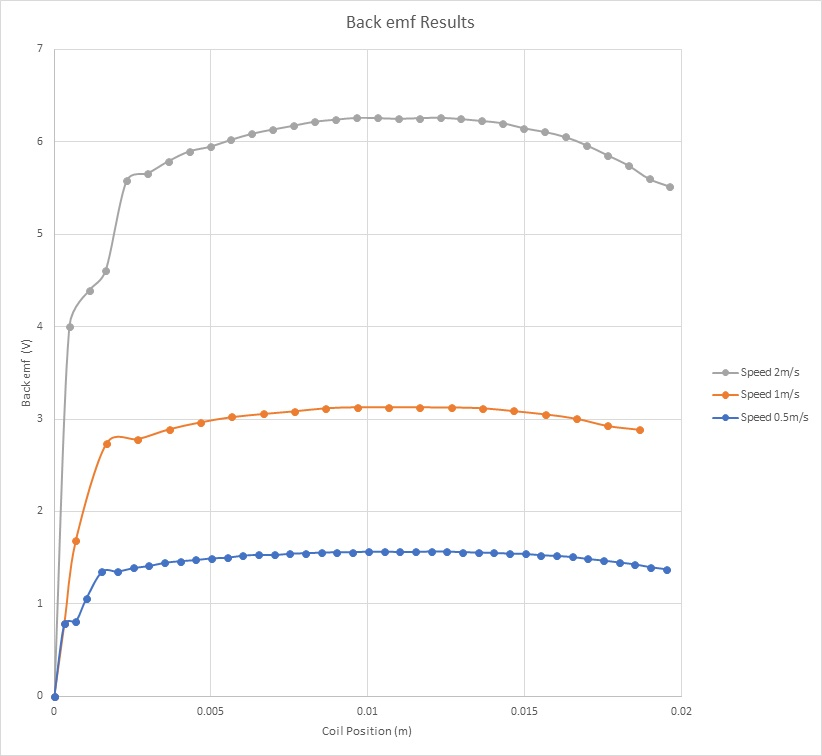Figure 8 - Back emf results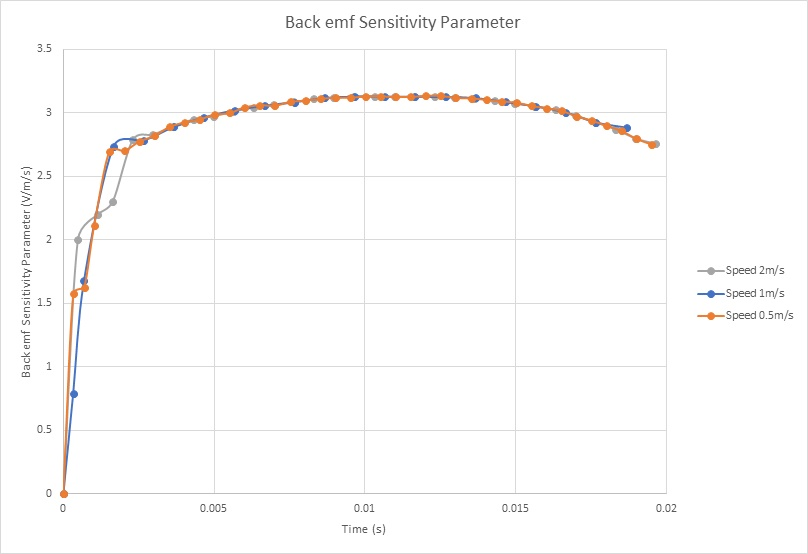Figure 9 - Back emf sensitivity parameter

Table 1 - Contains the main characteristics and properties of the studied voice coil actuator. EMS and experimental results are in good agreement.
 EMS Experimental Current +10 A-10A +10A-10A Resistance 20.3520.5Inductance 5mH 5mH Maximum Generated Force 34N 33.4N Force Sensitivity Parameter 3.2N/amp 3.1N/amp Back emf Sensitivity Parameter 3.1V/m/s 3.1V/m/s

Electro-Mechanical Simulation using EMS Coupled to SOLIDWORKS Motion – Coil displacement, speed, acceleration and current calculation

In this section, electrodynamic simulation is established to calculate and generate electromechanical results including magnetic fields, current, coil position, speed and acceleration, etc. Eddy current effect caused by motion is neglected. EMS coupled to SOLIDWORKS motion analysis is used to run these simulations. Figures 10a) contains a plot of the magnetic flux density at 20ms and 20V. It shows that the maximum field (1.77T) is located at the thin walls of the iron shell. At 20ms, both Current and position of the coil are 0.88A and 4.78mm, respectively. Figure 10b) gives a plot of the magnetic flux at 9ms and 200V. The peak values of the magnetic flux are about 2T and reached in the iron shell. The coil achieves a position of 8.6 mm and a current of 9.26A at this instant. Magnetic fields animation are shown in Figures 11a) and 11b).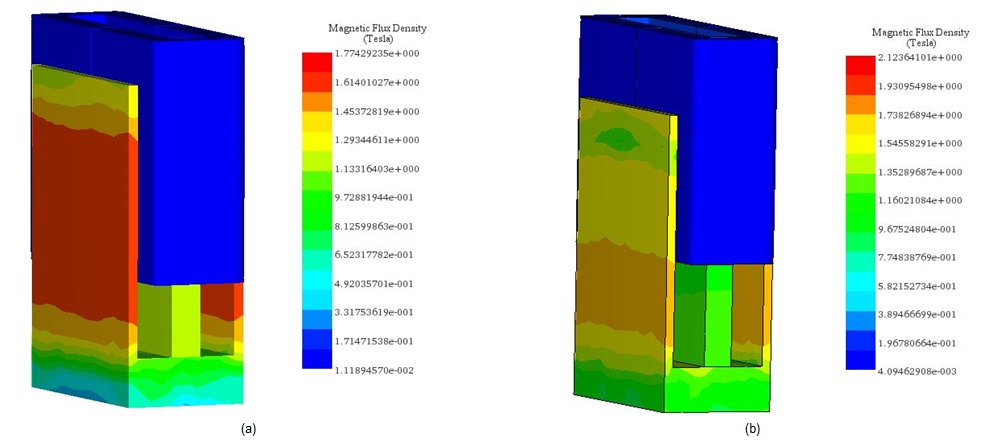Figure 10 - Magnetic flux density results, a) in case of 20V, b) in case of 200V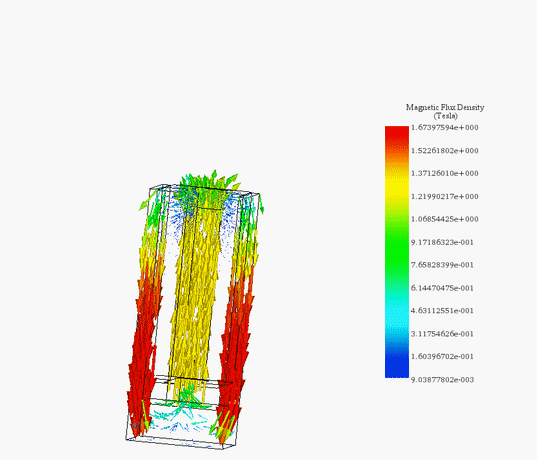(a)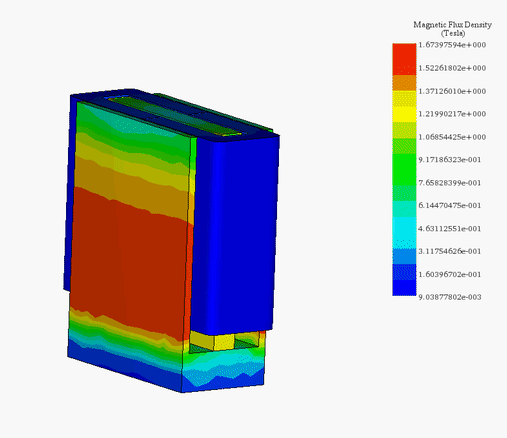(b)
Figure 11 - Animation plots of the magnetic flux, a) vector plot (200V) , b) fringe plot (20V)

Figures 12a) and 12b) and 12c) display the results of coil linear displacement, speed and acceleration for different applied voltages. For a DC voltage of 20V, the voice coil actuator moves from 0 mm at 0s to 4.7mm at 20ms. The maximum reached speed by the coil is nearly 0.5 m/s while the acceleration is about 30 m/s^2. When a 200V DC voltage is applied to the actuator, the moving coil goes from 0 mm to 20 mm in 13ms. This leads to a maximum speed and acceleration of 3.3 m/s and 308 m/s^2, correspondingly. The higher the voltage, the higher is the speed. This can be explained by Figure 13. Lorentz force increases with the current which is directly proportional to the applied voltage. The computed currents in the coil are 9.5A and 0.9A when the supplied voltages are respectively 200V and 20V.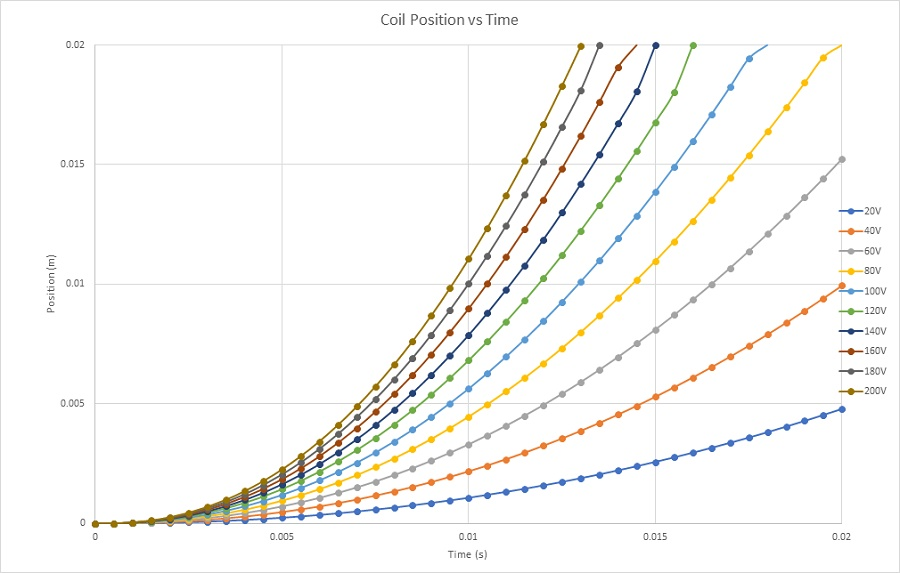Figure 12a - Coil position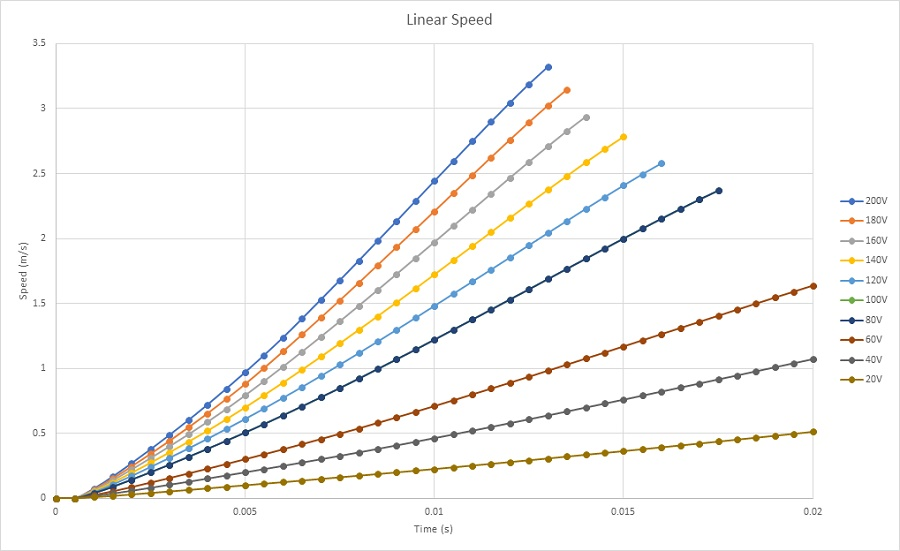Figure 12b - Coil speed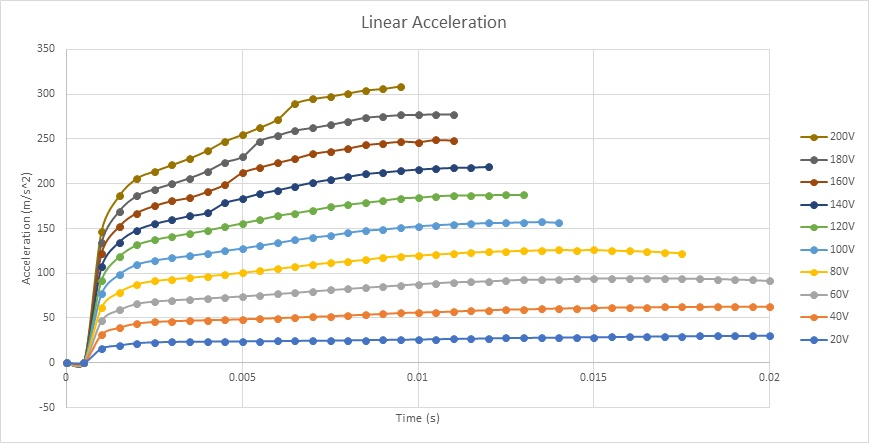Figure 12c - Coil acceleration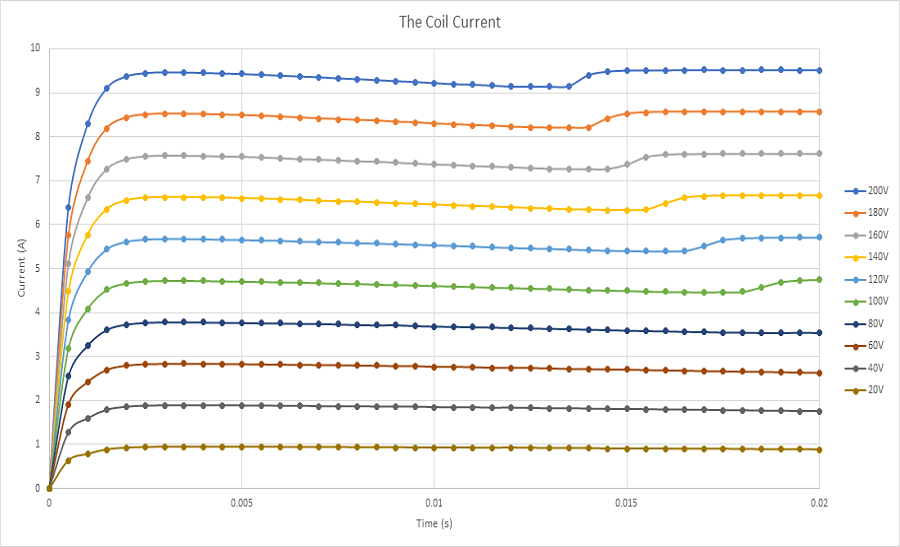Figure 13 - Currents results

## Conclusion

The voice coil actuator studied in this application showed many advantages such as constant force along the stroke, high speed and acceleration, etc. Computer simulation results demonstrates very good agreement with experimental and testing data. This will allow to create and build innovative voice coil actuators with improved efficiency and lower cost.

#### References

: Vahid Mashatan. Design and Development of an Actuation System for the Synchronized Segmentally Interchanging Pulley Transmission System (SSIPTS). Department of Mechanical and Industrial Engineering University of Toronto 2013